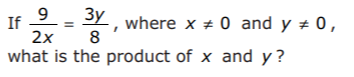1Evaluate your knowledge by attempting the following questions.  Ready? Fasten your seat belt!

$latex x=frac{y+1}{z-2}$

$latex ihbarfrac{partial}{partial t}left|Psi(t)right>=Hleft|Psi(t)right>$

x + √{y+3}=?

(x+y)/(y+5)